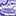# QlikView App Dev

Discussion Board for collaboration related to QlikView App Development.

Announcements
Skip the ticket, Chat with Qlik Support instead for instant assistance.
cancel
Showing results for
Did you mean:Not applicable

## Partial Sums Queries

Dear All,

I have two problems with the partial sums in a pivot table i am working on.

Firstly, in my pivot table, i'm using a grand total and 3 sub totals, however i only really need one sub total. Is there any way where i could hide or only calculate one sub total?

Secondly, when i make a selection in a listbox, the pivot table retracts and shows me the correct data relating to my selection in the list however, it changes the total to show only that of my selection. for example; i have 4 produts totalling 800, 200 each, so when i make the selection, the total will show me 200 instead of 800. How can this be solved?

This is a sample i obtained. http://community.qlik.com/members/msadrikhcb/files/totals-problem.qvw.aspx

I only need the total of functions of process 1 and the the grand total. Also, choosing a process from the listbox changes the grand total and thus the Agg.

PS. Since i pull data from a local server, i cannot use macros, share the .qvw file or edit script.

Best Regards,

1 Solution

Accepted SolutionsNot applicable
Author

Hi All,

Thanks for your answers and help, through trial and error and with the help of some other users, I have managed to find the following solution.

If(Dimensionality()>1, If(Product='A' OR Product='B' OR Product='C', If(trim([Month])='Jan', If(Product='A' OR Product='B' OR Product='C' )))

For the first problem we can implement dimensionality ans use the following;

This will solve the problem in pivot table to restrict partial sums.

For the second problem, we can use a text box or an input box thus creating a variable.

So instead of using Sum(Amount), we use Sum({\$} Amount). This in a variable or a text box will display the Total amount yet when aselection is made the total will remain the same i.e. if we need to show 800 and we choose the product which is 200 units then the unit will be displayed as 200 but the total will return the 800.

4 RepliesNot applicable
Author

Your first problem I think it´s easy to solve. Just go presentation tab in pivot table properties and you will control subtotals by marking or unmarking partial sums option (by each dimension).

The second one I think it has no solution because it´s qlikview standard functionality. Depending on what you are trying to do, you must create another figure to solve your problem. In my work, I use a lot of pivot tables but that functionality isn´t a problem for me; if I don´t want to lose some data, I use a text box to do some calculations.

However, if you look at the finance controlling example on your qlikview, you can collect some ideas.

I hope It hepls you.

FiberNot applicable
Author

Hi Fiber,

Thanks for your answer, but it's not what i was looking for. The subtotals come under the expressions so i cannot uncheck them and besides i need one out of the three, in presentation tab all of them are either checked together or not at all.

With regard to the second, i will try to use a seperate text box.

Regards,Partner - Creator II

First Solution,

Calculate the sum of process on scripting part with if condition.

so u will get the one sub total insted of three sub total.Not applicable
Author

Hi All,

Thanks for your answers and help, through trial and error and with the help of some other users, I have managed to find the following solution.

If(Dimensionality()>1, If(Product='A' OR Product='B' OR Product='C', If(trim([Month])='Jan', If(Product='A' OR Product='B' OR Product='C' )))

For the first problem we can implement dimensionality ans use the following;

This will solve the problem in pivot table to restrict partial sums.

For the second problem, we can use a text box or an input box thus creating a variable.

So instead of using Sum(Amount), we use Sum({\$} Amount). This in a variable or a text box will display the Total amount yet when aselection is made the total will remain the same i.e. if we need to show 800 and we choose the product which is 200 units then the unit will be displayed as 200 but the total will return the 800.Community Browser